Published

# Arduino Based Parking Assistant V2

In this video, I am going to use an ultrasonic sensor to calculate the car's distance from the garage wall and display it using green, blue,

BeginnerProtip1 hour6,475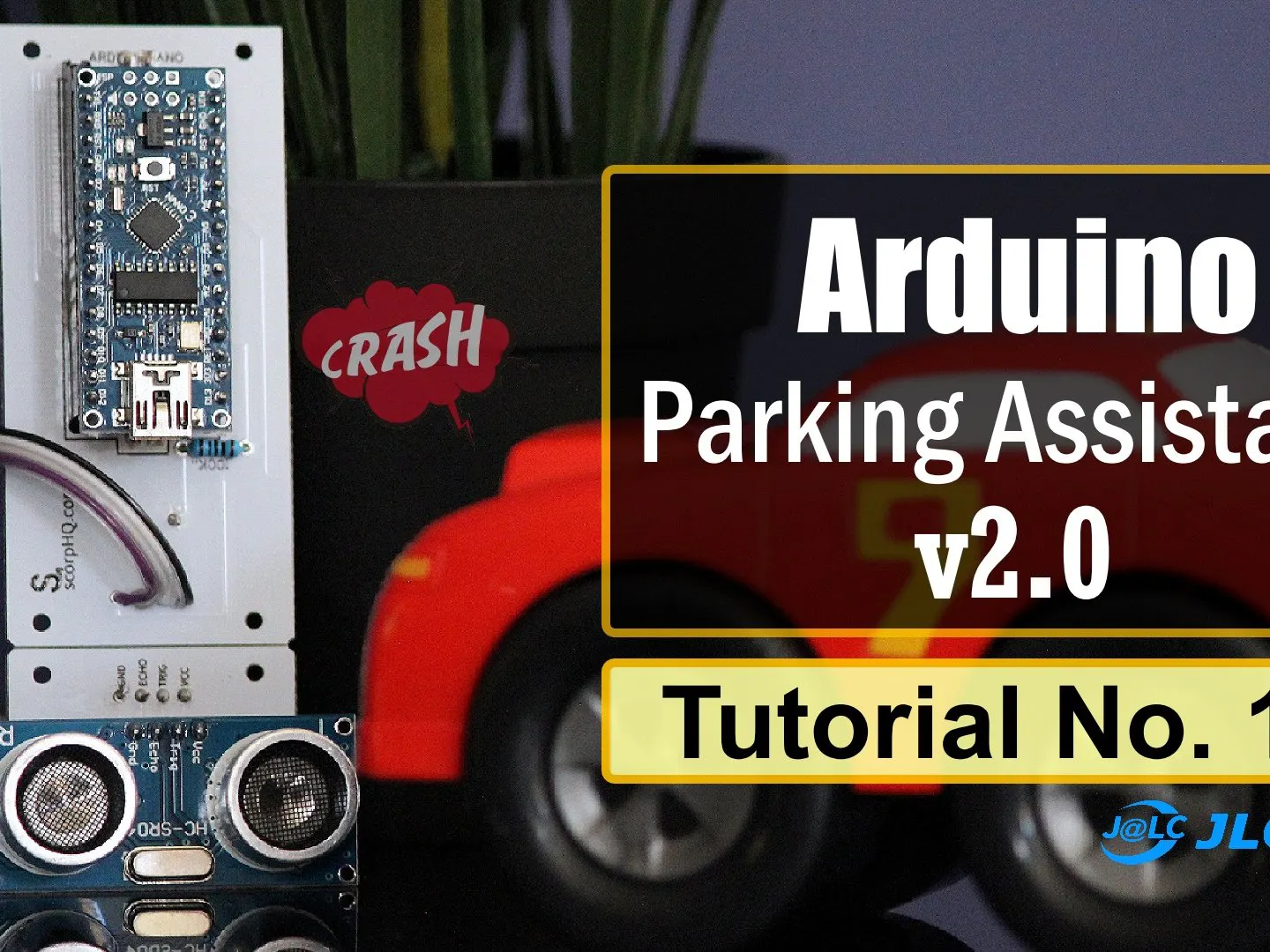## Things used in this project

### Hardware components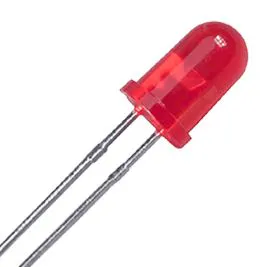DIYables LED
×8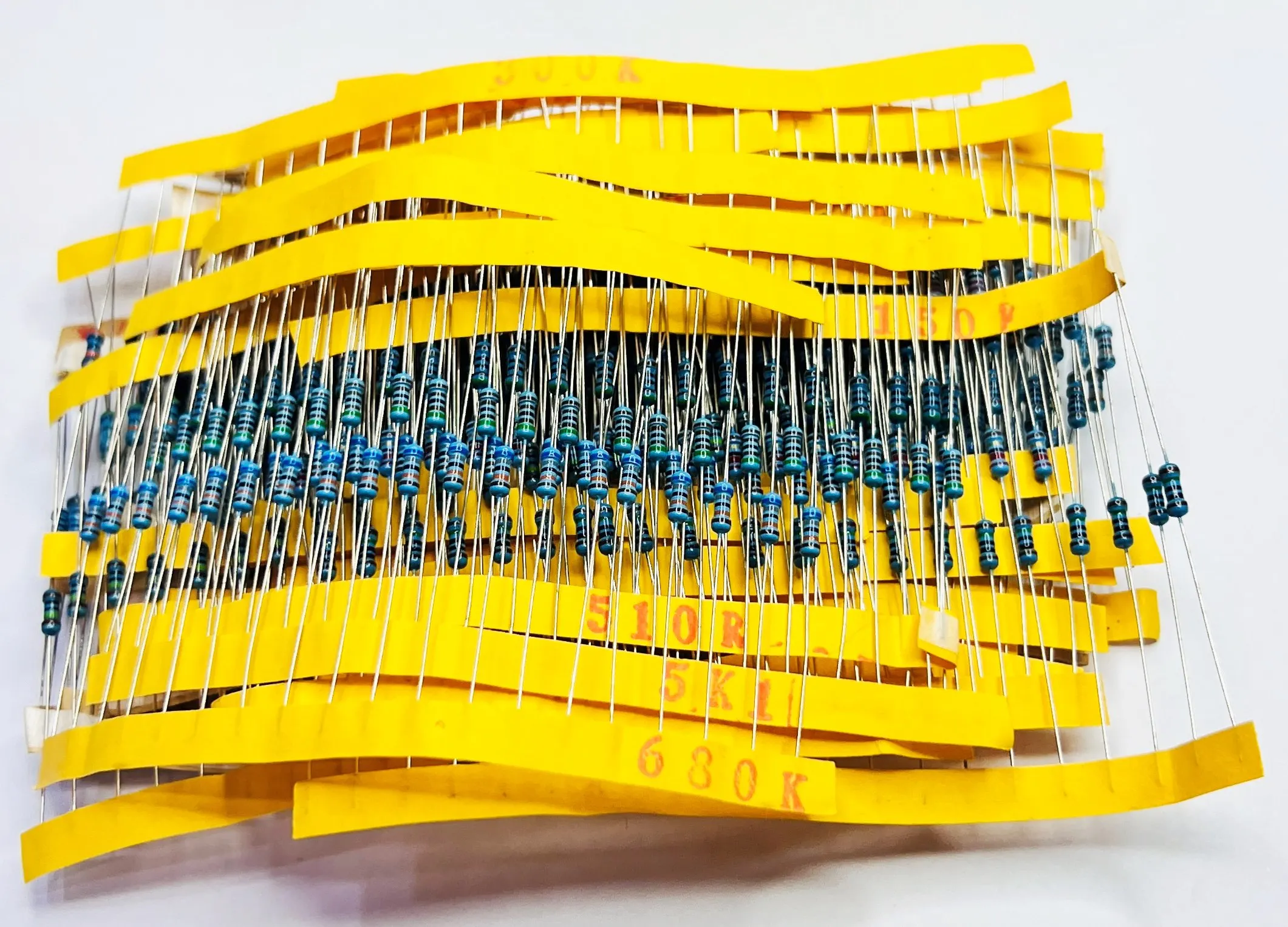DIYables Resistor
×8Arduino Nano R3
×1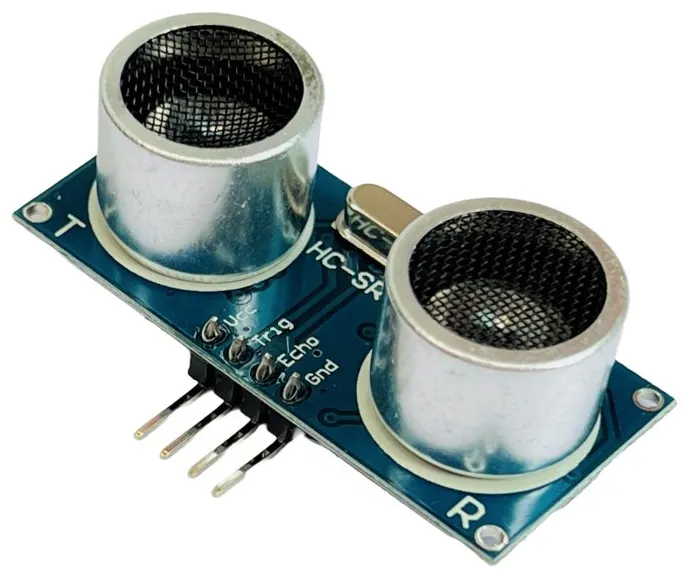DIYables Ultrasonic Sensor HC-SR04
×1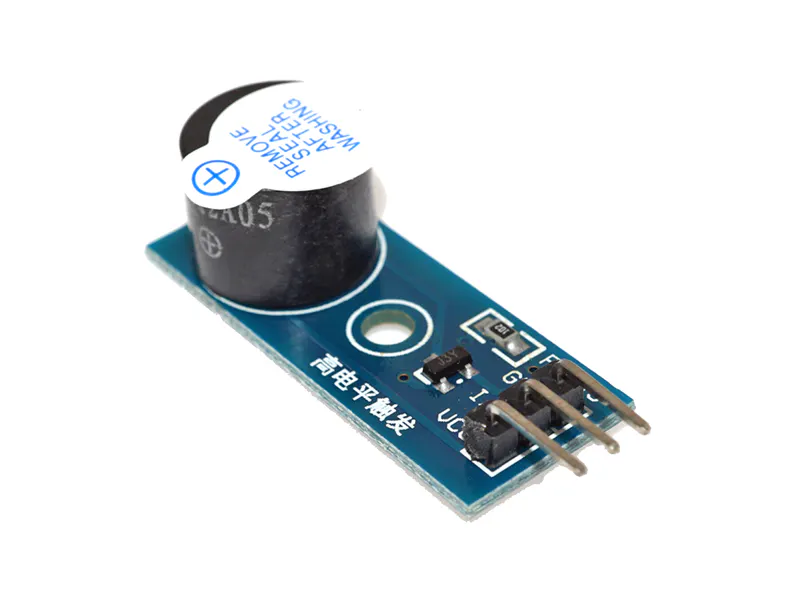DIYables Buzzer
×1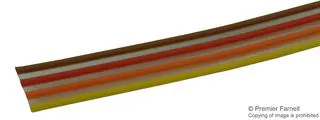Ribbon Cable, Color Coded Flat
×1DIYables USB Cable for Arduino Nano
×1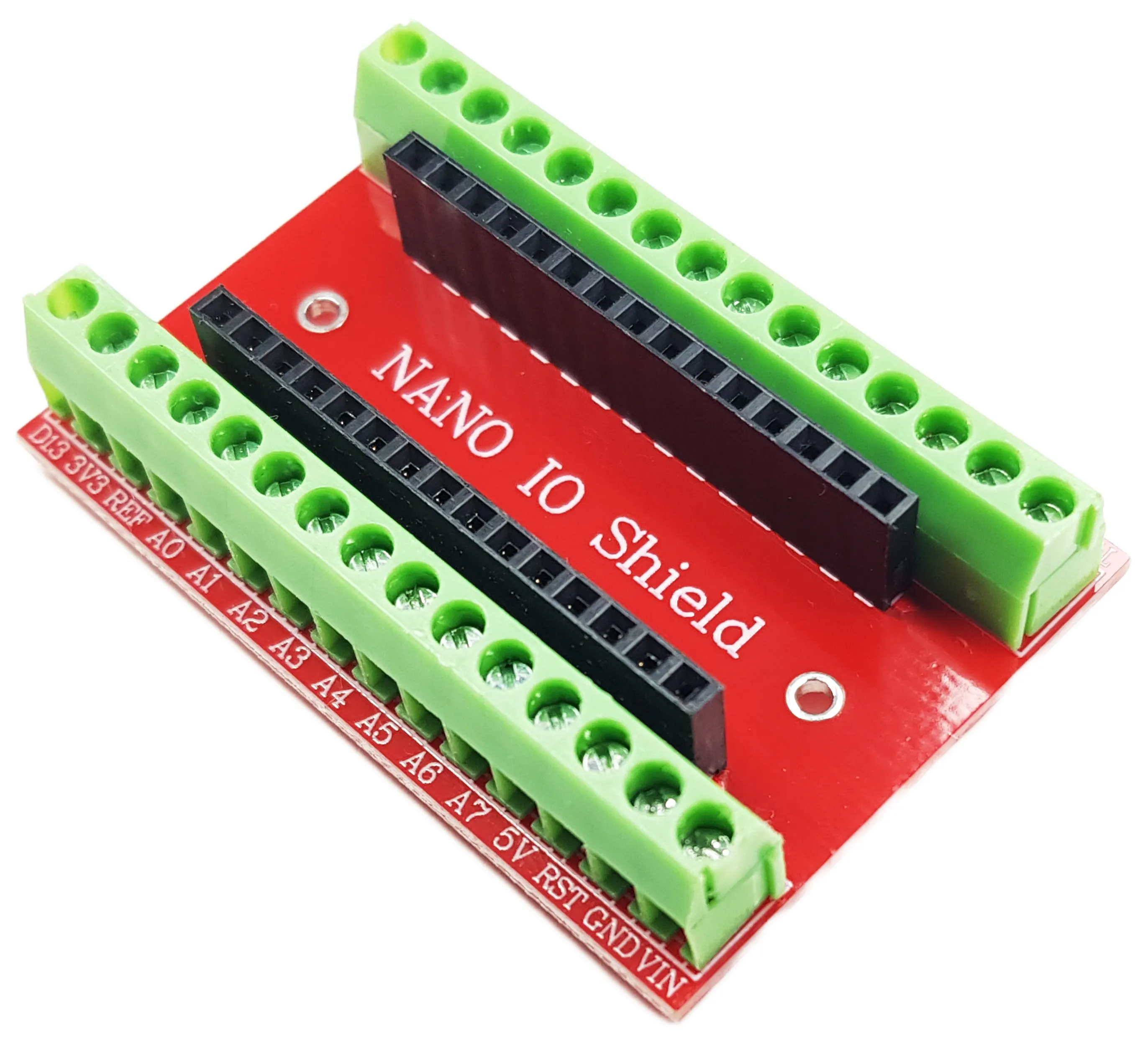DIYables Screw Terminal Shield For Arduino Nano
×1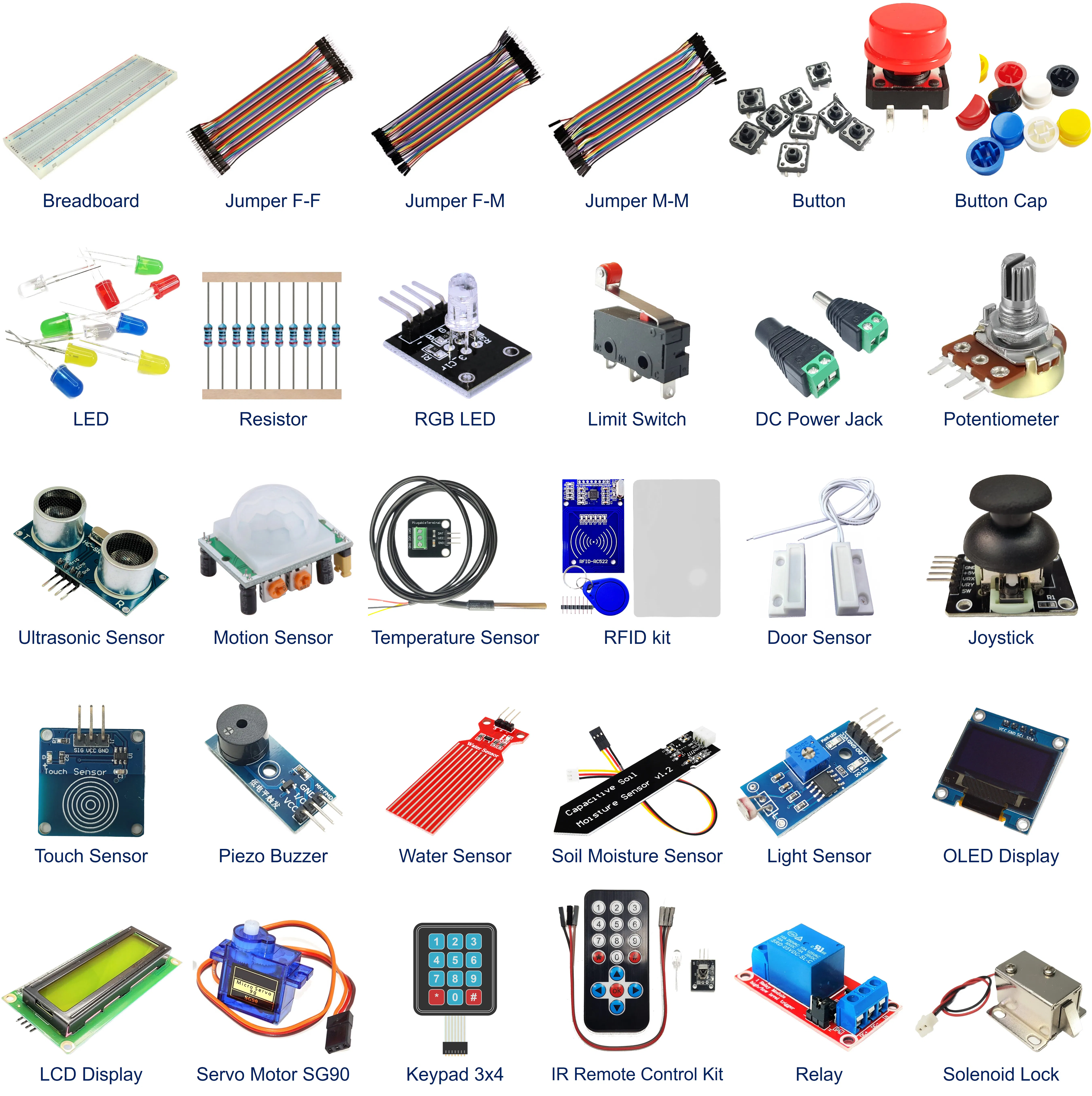DIYables Starter Kit
×1

## Schematics

### Schematic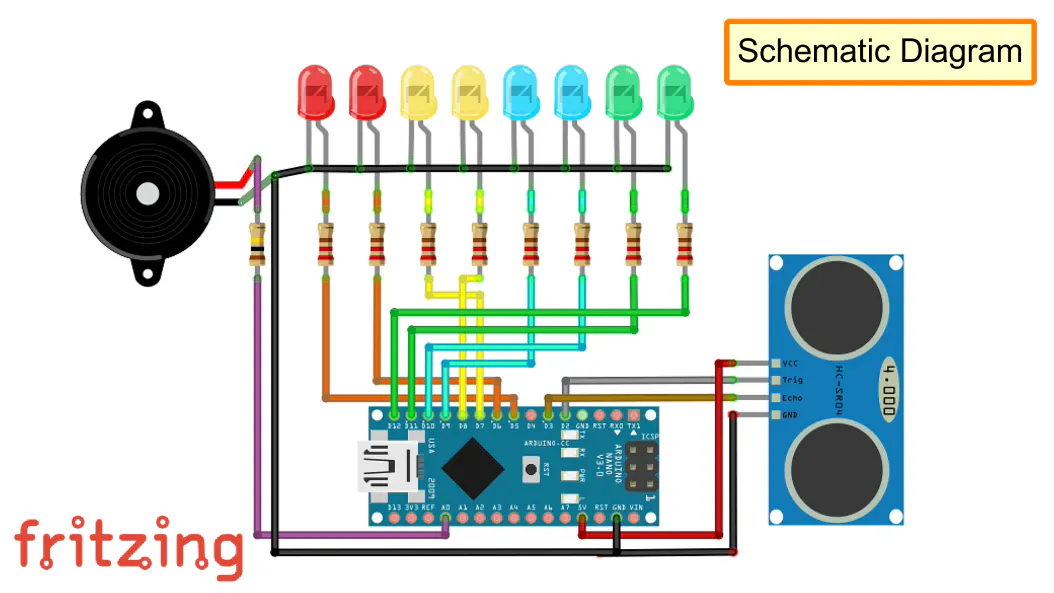### Sketch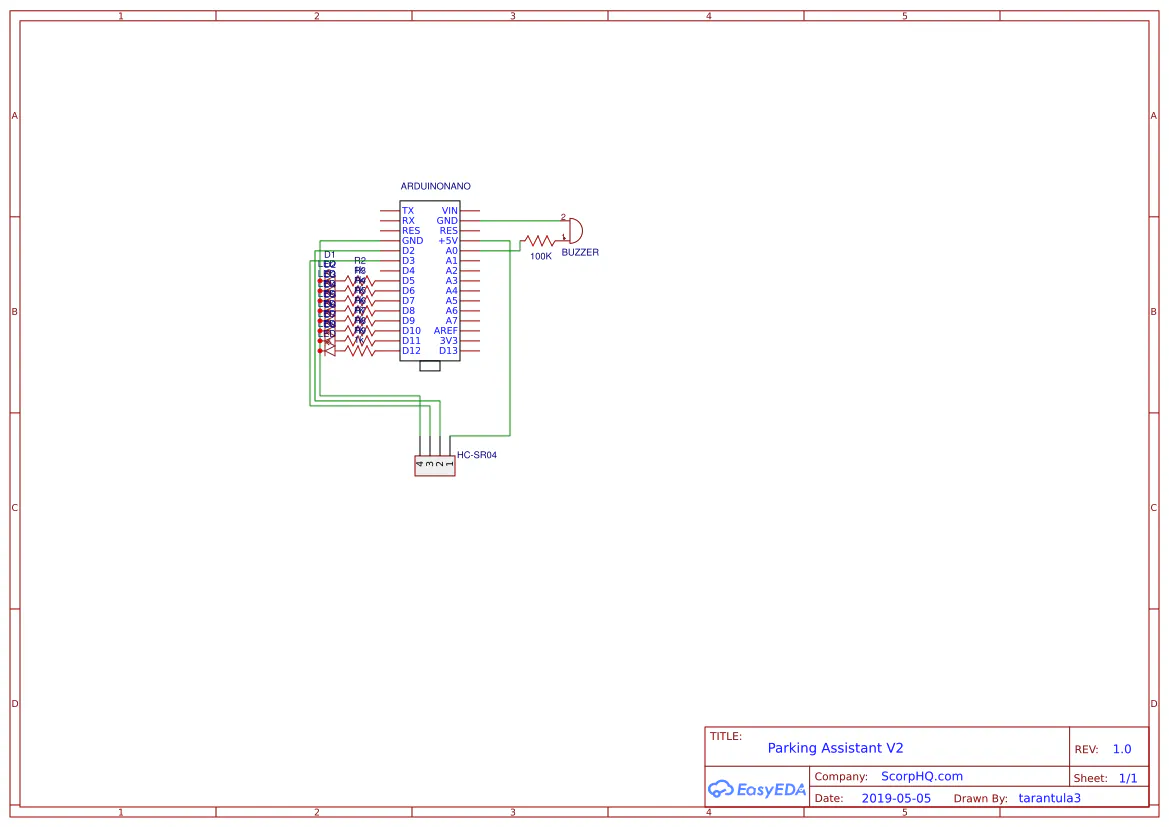## Code

### Untitled file

Arduino
```#include <NewTone.h>

int trigPin = 2; // Sensor Trip pin connected to Arduino pin D5
int echoPin = 3; // Sensor Echo pin connected to Arduino pin D6
int r1LED  = 5;
int r2LED  = 6;
int y1LED  = 7;
int y2LED  = 8;
int b1LED  = 9;
int b2LED  = 10;
int g1LED  = 11;
int g2LED  = 12;
int buzzer = A0; // Buzzer connected to Analogue pin A0
long TempDistance = 0; // A variable to store the temporary distance
int counter = 0; // Counter value to check if the object has stopped moving

void setup() {
Serial.begin(115200);
pinMode(trigPin, OUTPUT);
pinMode(echoPin, INPUT);
pinMode(r1LED,   OUTPUT);
pinMode(r2LED,   OUTPUT);
pinMode(y1LED,   OUTPUT);
pinMode(y2LED,   OUTPUT);
pinMode(b1LED,   OUTPUT);
pinMode(b2LED,   OUTPUT);
pinMode(g1LED,   OUTPUT);
pinMode(g2LED,   OUTPUT);
pinMode(buzzer,  OUTPUT);
}

void loop() {
long duration, Distance;
digitalWrite(trigPin, LOW);   delayMicroseconds(2);
digitalWrite(trigPin, HIGH);  delayMicroseconds(10);
digitalWrite(trigPin, LOW);
duration = pulseIn(echoPin, HIGH);
Distance = (duration/2) / 74; // Distance in Inches

if(counter < 20){ // Do the rest if the car is still moving
if (Distance > 200) { turnThemAllOff(); } // Nothing in the garrage
if (Distance <= 110){ digitalWrite(g2LED, HIGH); } else { digitalWrite(g2LED, LOW); } // Turn on the 1st Green LED
if (Distance <= 80) { digitalWrite(g1LED, HIGH); } else { digitalWrite(g1LED, LOW); } // Turn on the 2nd Green LED
if (Distance <= 70) { digitalWrite(b2LED, HIGH); } else { digitalWrite(b2LED, LOW); } // Turn on the 1st Blue LED
if (Distance <= 60) { digitalWrite(b1LED, HIGH); } else { digitalWrite(b1LED, LOW); } // Turn on the 2nd Blue LED
if (Distance <= 50) { digitalWrite(y2LED, HIGH); } else { digitalWrite(y2LED, LOW); } // Turn on the 1st Yellow LED
if (Distance <= 40) { digitalWrite(y1LED, HIGH); } else { digitalWrite(y1LED, LOW); } // Turn on the 2nd Yellow LED
if (Distance <= 30) { digitalWrite(r2LED, HIGH); } else { digitalWrite(r2LED, LOW); } // Turn on the 1st Red LED
if (Distance <= 20) { digitalWrite(r1LED, HIGH); } else { digitalWrite(r1LED, LOW); } // Turn on the 2nd Red LED
if (Distance <  10) { NewTone(buzzer, 500); }      else { noNewTone(buzzer); }        // Item is way to close - Start the buzzer
delay(500);
};

if ((Distance == TempDistance) || ((Distance+1) == TempDistance) || ((Distance-1) == TempDistance)){
if(counter >= 20){ // Turn off the lights if the object hasn't moved for 20 cycles (no change in distance)
Serial.println("No movement detected, turning off the lights");
turnThemAllOff();
} else { counter++;   };
} else   { counter = 0; }; // Reset counter if there is a movement

TempDistance = Distance;
Serial.print(Distance);     Serial.println(" inches");
Serial.print("Counter : "); Serial.println(counter);
}

// Function to turn the LEDs off
void turnThemAllOff(){
digitalWrite(r1LED, LOW);
digitalWrite(r2LED, LOW);
digitalWrite(y1LED, LOW);
digitalWrite(y2LED, LOW);
digitalWrite(b1LED, LOW);
digitalWrite(b2LED, LOW);
digitalWrite(g1LED, LOW);
digitalWrite(g2LED, LOW);
noNewTone(buzzer);
}
```

## Credits

### Tarantula3

57 projects • 78 followers
There were 1000+ sperms but I was the fastest one..

### DIYables

0 projects • 36 followers
I would like to invite you to join and add your projects to DIYables platform https://www.hackster.io/diyables